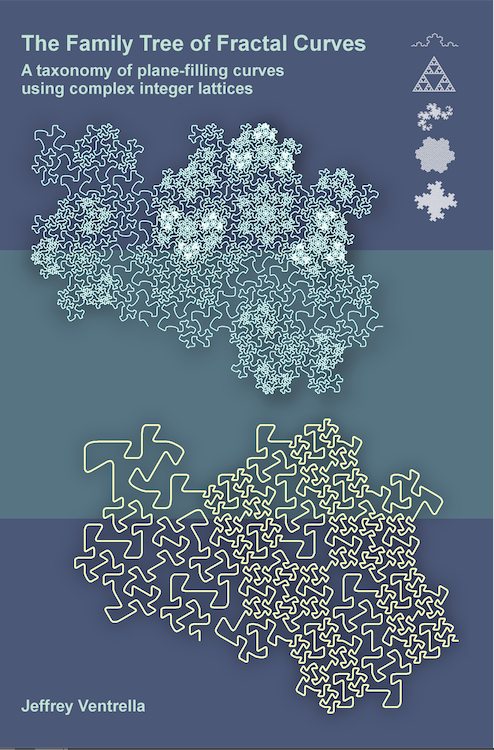The Family Tree of Fractal Curves A taxonomy of plane-filling curves using complex integer lattices by Jeffrey Ventrella 1 Introduction 2 Taxonomy 3 Iteration 4 Morphology 5 Family Resemblances 6 Undiscovered Curiosities 7 References

 5. Family Resemblances Fractal curves exhibit data amplification upon iteration of their relatively simple genetic origins. There is wonderful diversity among the plane-filling curves. And there are also obvious similarities. These curves—being constrained by the integer lattice—have certain properties that can be described algebraically. Many of the resemblances among curves are determined by where they lie within their family hierarchies—and some of these resemblances can be characterized using integer math. Primes, Composites, Roots, and Powers Prime numbers are fundamental in multiplication. They are the primitive building blocks of divisibility. Curves of prime root families are likewise fundamental. For instance, prime curves can only have integers that are units. The product of any two integers with norms > 1 is a composite integer. Composite root families have curves that are identical to the curves of their associated divisor families (except they are larger, and possibly rotated). Consider the rational integer 10: 2 and 5 are its divisors. Similarly, all of the curves in the G(1,1) family and all of the curves in the G(2,1) family can be found in the G(3,1) family, which is a composite root family. Figure 5.1 shows three curves of the G(3,1) family with norm 5. The two at the bottom are identical to curves found in the G(1,1) and G(2,1) families, except they are larger and rotated. The curve shown at the top is unique only to the G(3,1) family (and its descendants/multiples).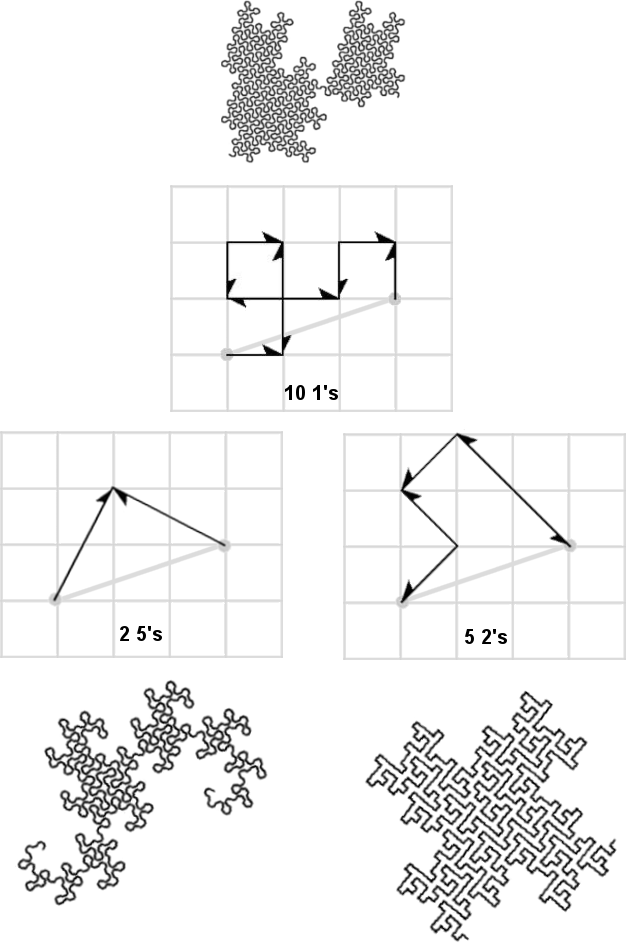Figure 5.1. The G(3,1) family has curves identical to those of the G(1,1) and G(2,1) families You could say the composite root families have a larger phenotype space than prime root families, since they possess curves that are isometric to the root curves of their constituent divisor families. Flesh-Creative Power Descendants When it comes to family resemblances in curves, exponentiation is more exciting than multiplication. The phenotype space is larger among power families. As you journey along a family set, self-similarity grows, not just in terms of the scaled-up repetition of divisor curves, but in the creative hybridizing of their ancestry. For instance, Figure 5.2 shows the beginning of the G(1,1)n family set. The HH dragon is shown at the root, with progressively more complex variations in the G(1,1)2 and G(1,1)3 families.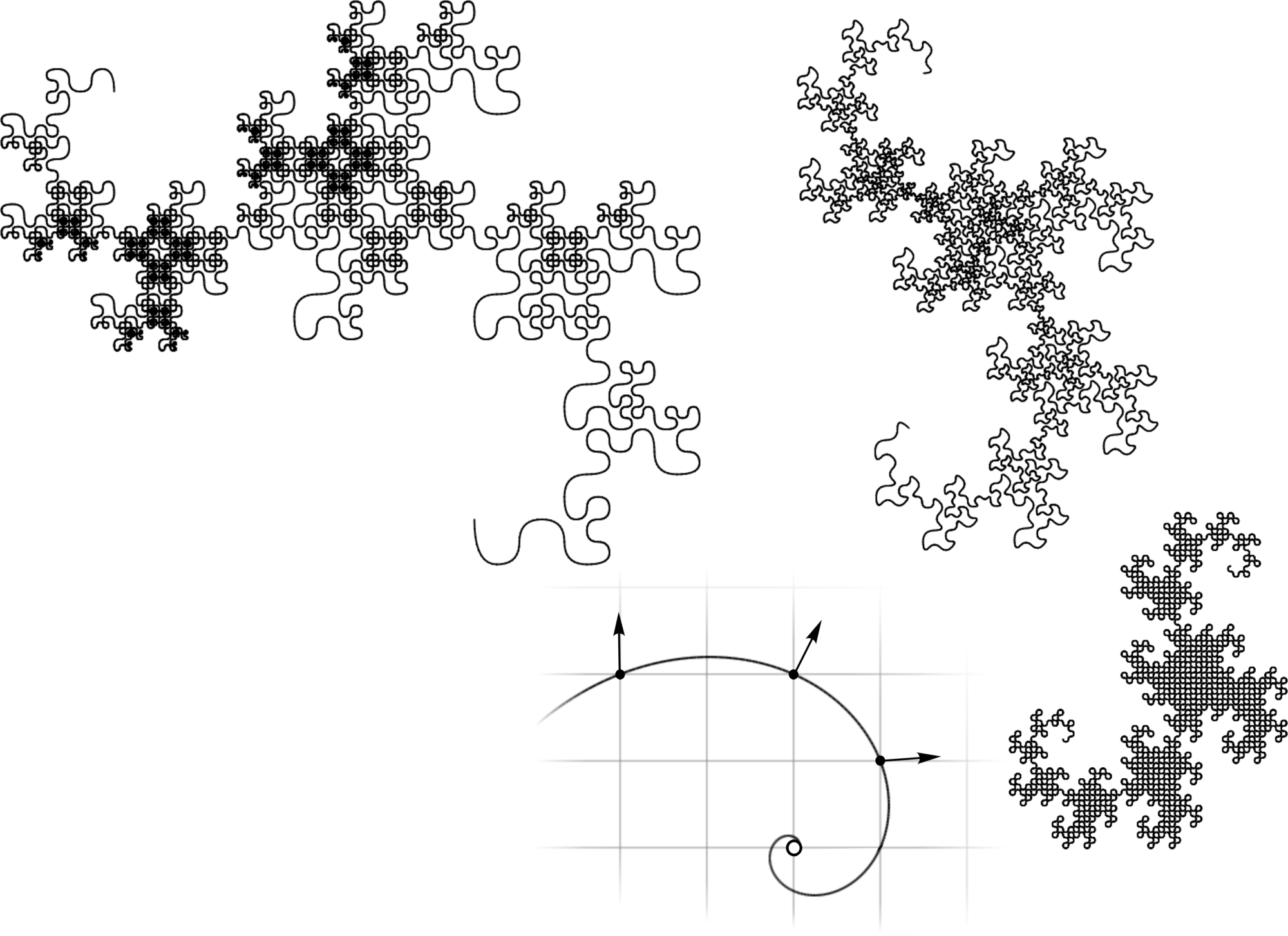Figure 5.2. The first three curves of the G(1,1)n family set descended from the HH Dragon There are apparently many more flesh-variations of the HH Dragon body shape along this family set. Figure 5.3 shows the HH dragon and six of its descendants. They are all splined and normalized to the space of the HH Dragon.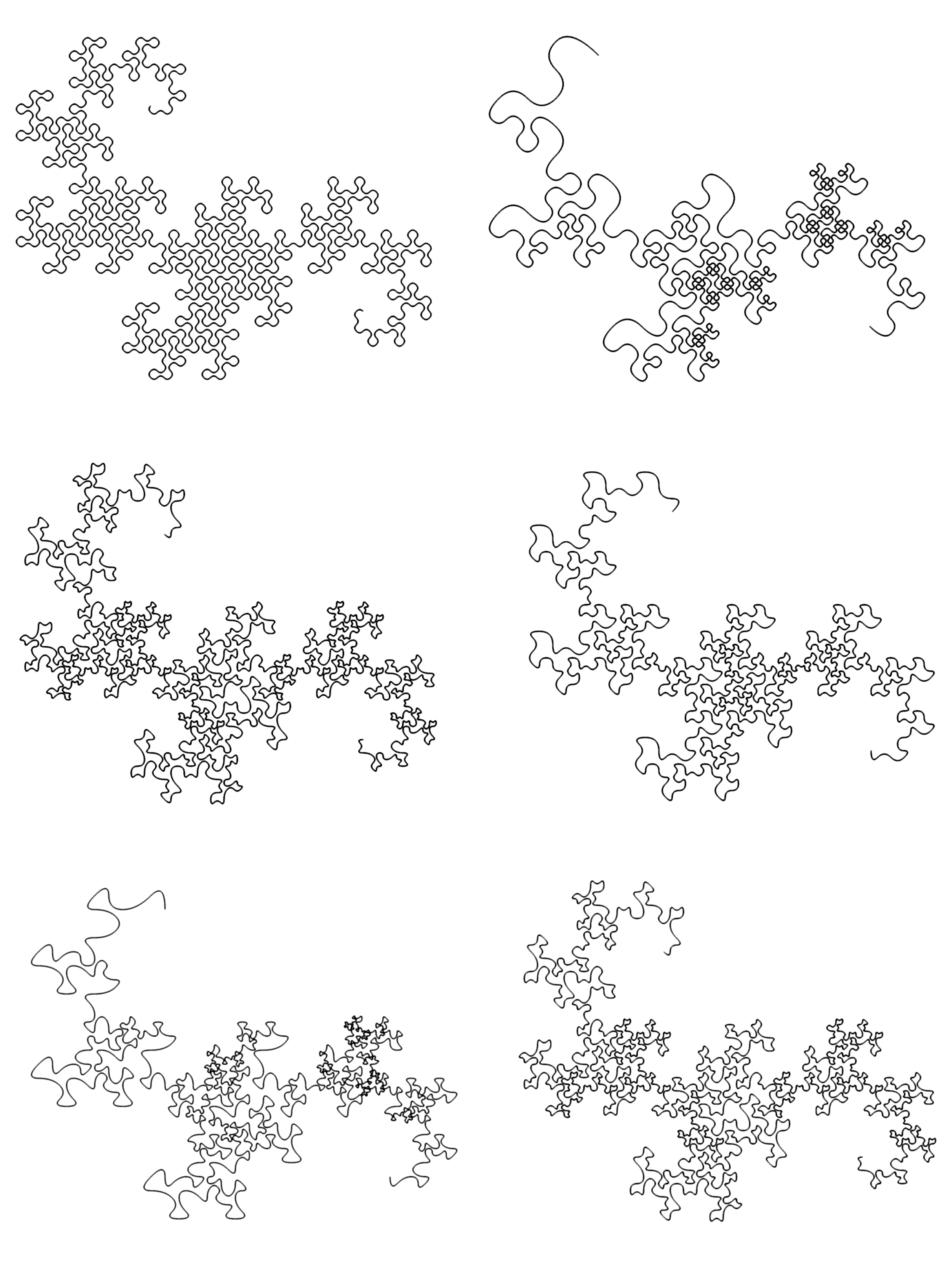Figure 5.3. The HH Dragon with five descendants in the G(1,1)n family set The variety of a family grows with exponentiation (relative to its order in the family set). In power families, many body shapes are isometric to those of their ancestors (in families closer to the start of the family set), but their flesh can be more complex, owing to the fact that they can have multiple-length segments. Figure 5.4 shows three curves of the E(2,1) family (left side) associated with more complex-flesh curves in the E(2,1)2 family (right side) that have the same body shape. Rotations and scales have been normalized to emphasize only differences in flesh.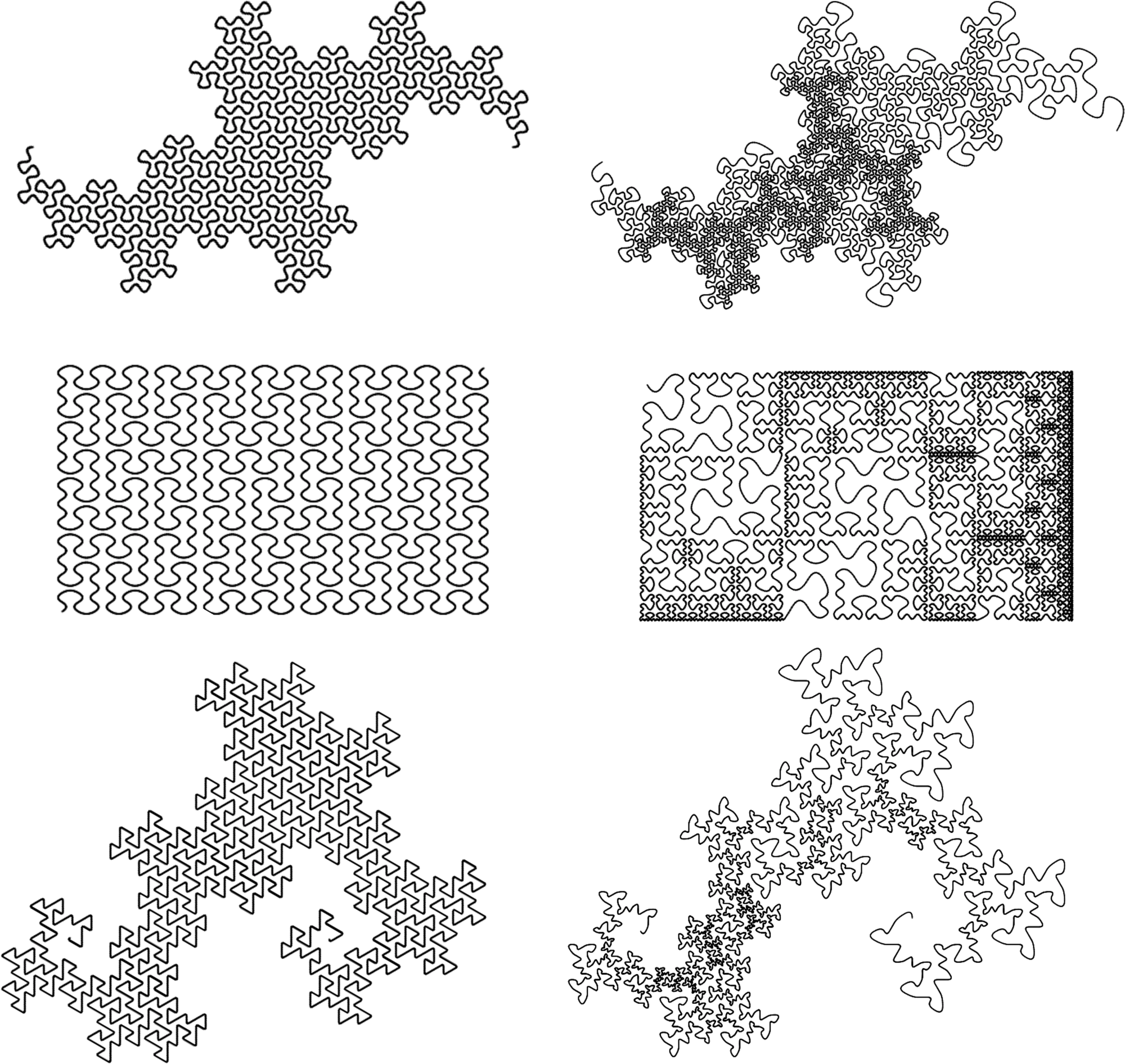Figure 5.4. Some curves in the E(2,1) family (left), shown with descendants (right) Another example of morphological similarity within a family set is shown in Figure 5.5. The first four teragons of the Dragon of Eve are shown in the left column. The other two columns show the first four teragons of related curves from the G(1,1)2 and G(1,1)3 families. They are normalized to the family space of the Dragon of Eve for comparison. Tergaon 4 of the curve from the G(1,1)3 family is shown larger at bottom-right (splined). Notice how the “horn” of the Dragon of Eve teragon has an extra protrusion added to make the other teragons. This has an effect not unlike iterating the teragon to the next order, but only doing it on the middle segment. Each of these curves are self-avoiding. Is it possible that similar protrusions could be added to the horns of consecutive relatives, resulting in more curly Dragons?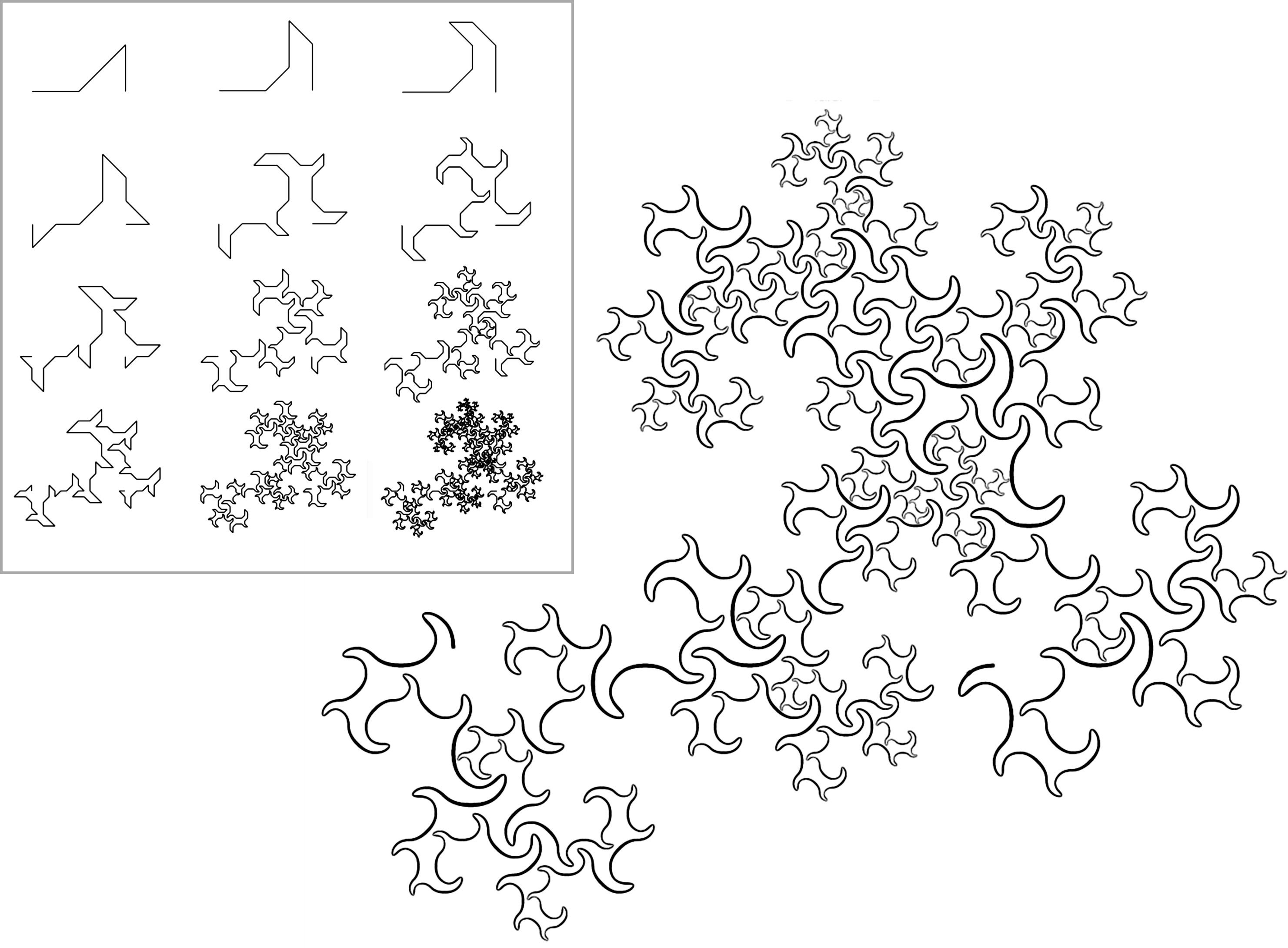Figure 5.5. Top: Dragon of Eve and relatives in the G(1,1)2 set; bottom: third relative A similar technique can be applied to Mandelbrot’s Quartet by representing it in the G(5,0) family with each integer having norm 5. One of its segments is replaced with a copy of the original generator, to create a more elaborate sweep (Figure 5.6).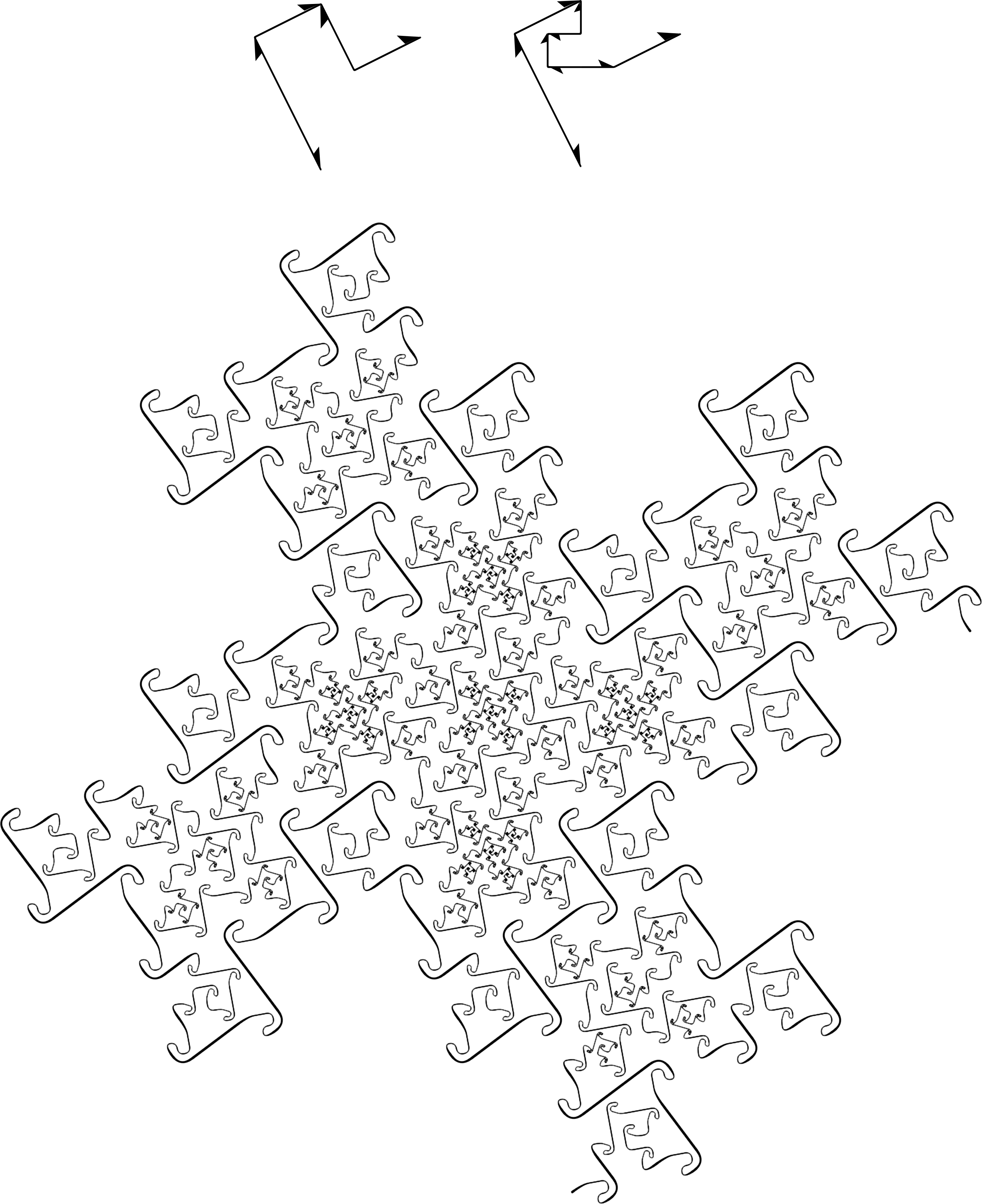Figure 5.6. Modified version of Mandelbrot’s Quartet, with stylized rendering Axis-aligned curves A subset of the complex integers (of both domains) is the set of rational integers. They lie on the real axis. Figure 5.7 shows the beginning of the positive side of this set in the Gaussian domain. The diagonal axis has norms that are twice those of the real axis.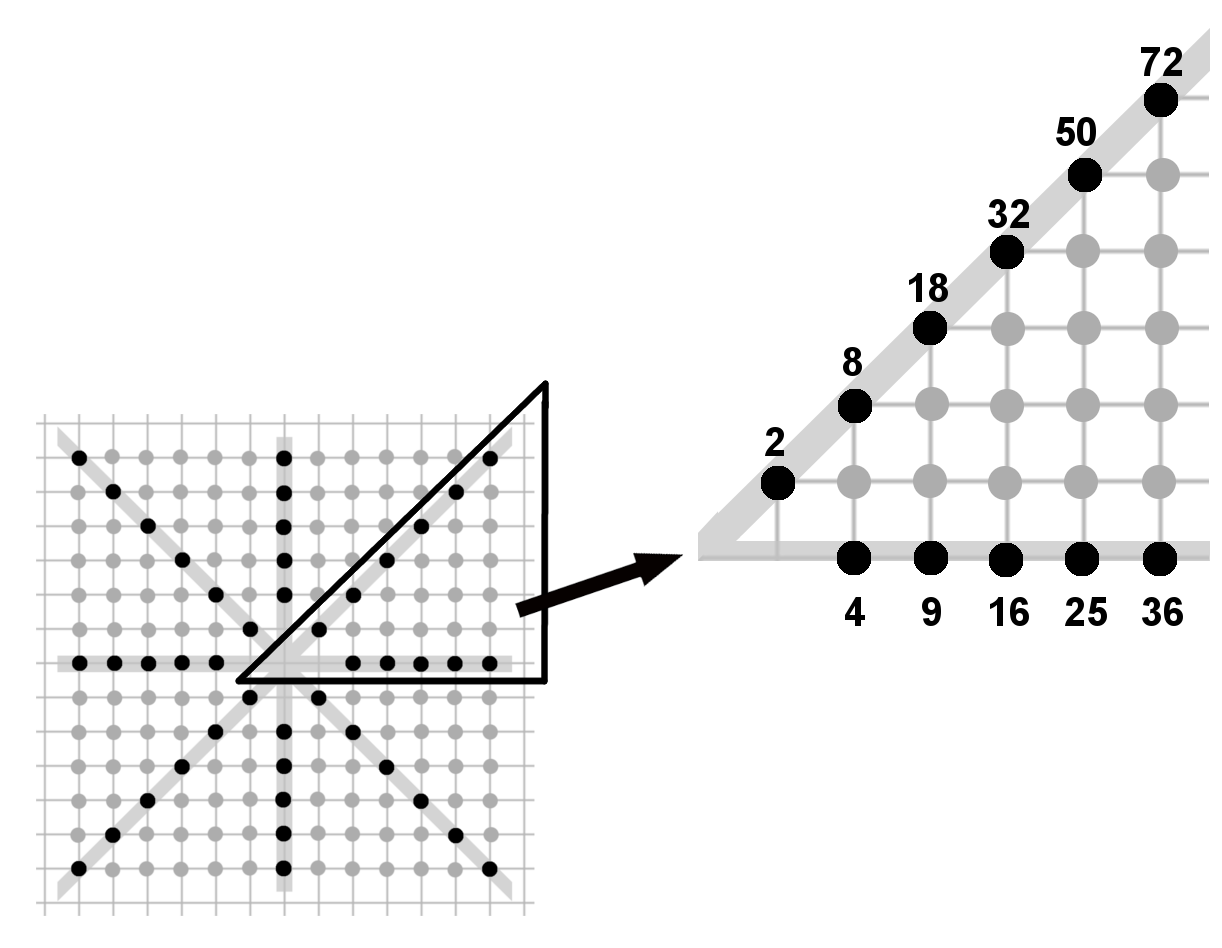Figure 5.7. Axis-aligned norms in the Gaussian domain Let’s refer to the orthogonal axes in Figure 5.7 as “primary axes,” and the diagonal axes as “secondary axes.” Each axis starts at the origin and extends out to infinity. The Gaussian domain has four primary (orthogonal) axes and four secondary (diagonal) axes. The Eisenstein domain has six primary axes and six secondary axes. Primary axes correspond to the directions of the units. Here’s an observation: the families that lie on these axes appear to be the only ones that include curves with straight-edge skins (skins with a fractal dimension of 1). For example, curves with square or equilateral triangle body shapes can only be found along the primary axes. Two such curves are shown in Figure 5.8 (splined).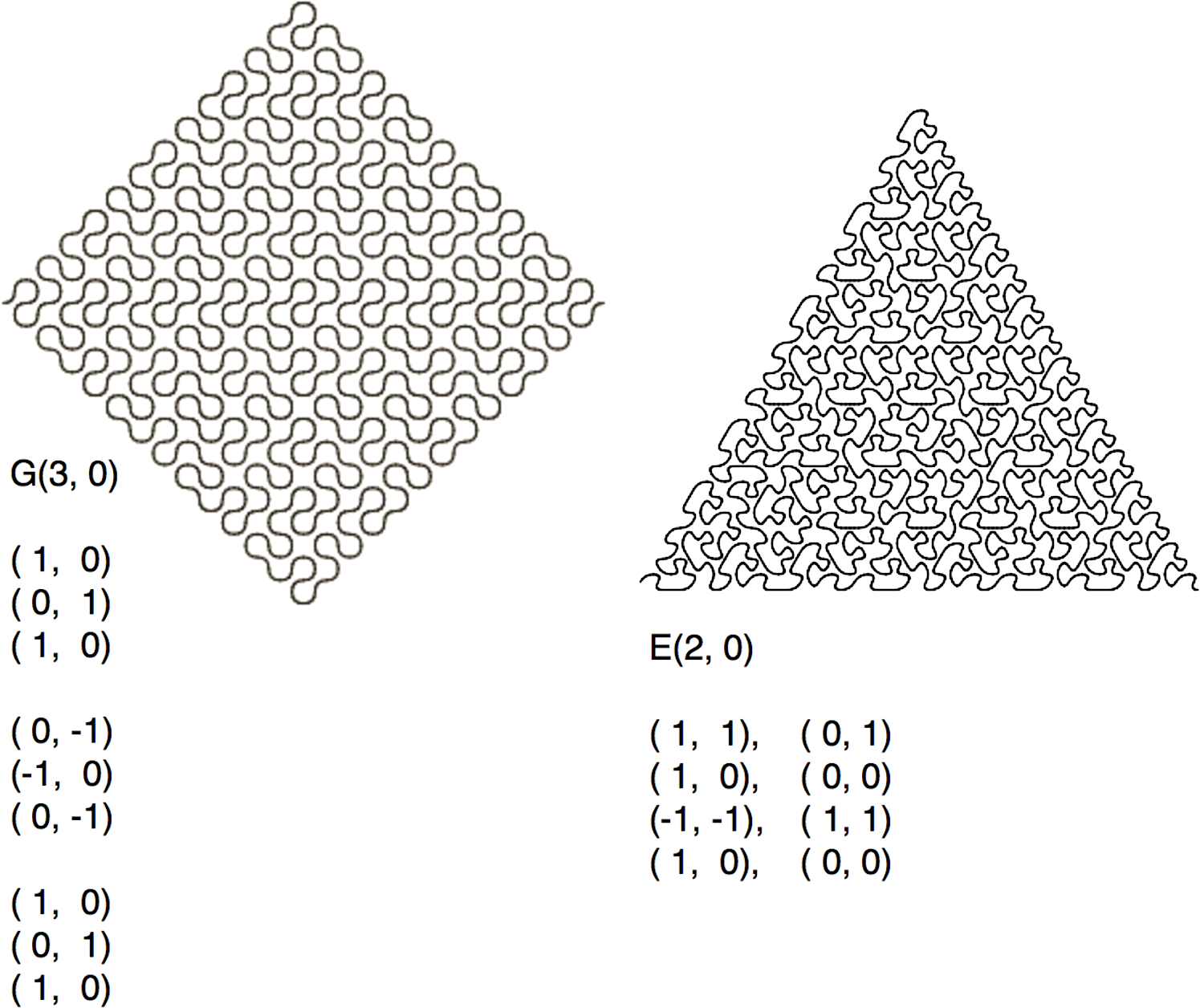Figure 5.8. Two (splined) curves with regular polygon boundaries The famous Sierpinski arrowhead curve is a gasket of the E(2,0) family, which lies on the primary axis. Like the example at the right in Figure 5.8, it occupies a regular triangle—but only partially, because its fractal dimension is only 1.5849 (Figure 5.9).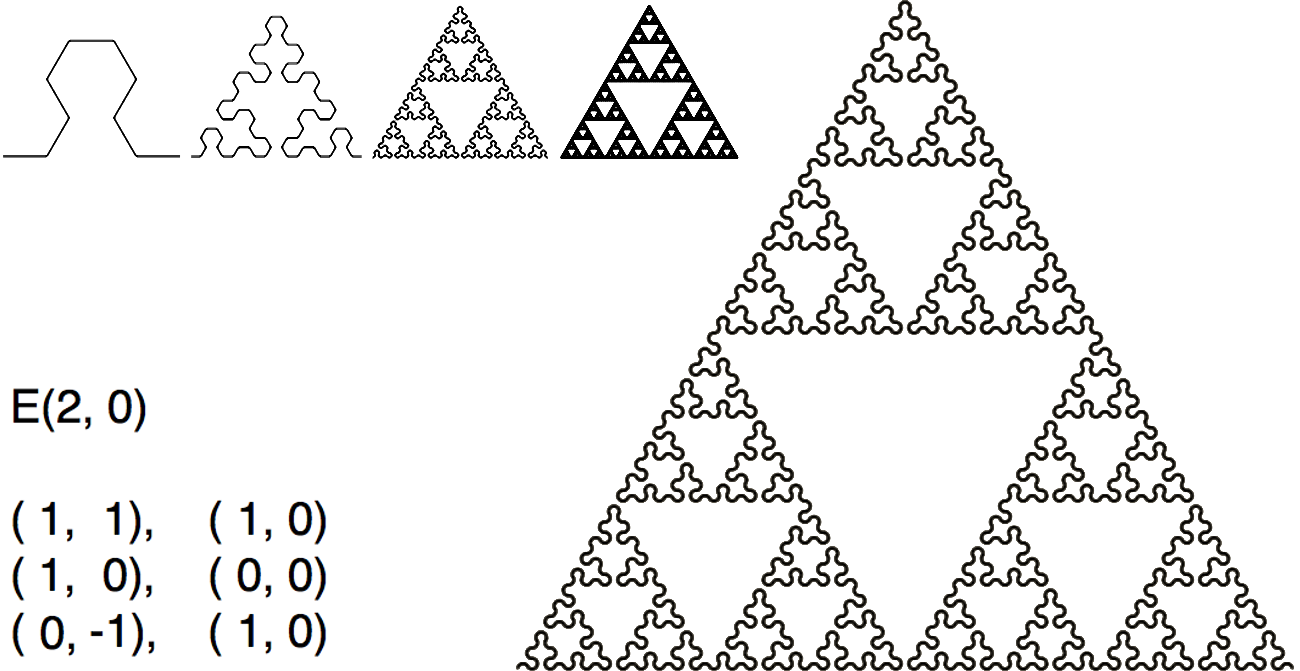Figure 5.9. The Sierpinski arrowhead curve Question: is the Sierpinski arrowhead curve self-avoiding? It would seem so, from looking at the first few teragons. However, at infinity, it is identical to the Sierpinski Gasket, which is an infinite number of infinitely small kissing triangles (Figure 5.10).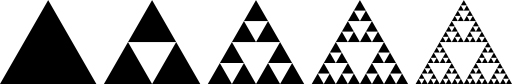Figure 5.10. The Sierpinski Gasket By the way: in the same family as the Sierpinski arrowhead curve (E(2,0)) is a curious relative, affectionately called “Sierpinski Family”. It has its own way of expressing self-similarity (Figure 5.11).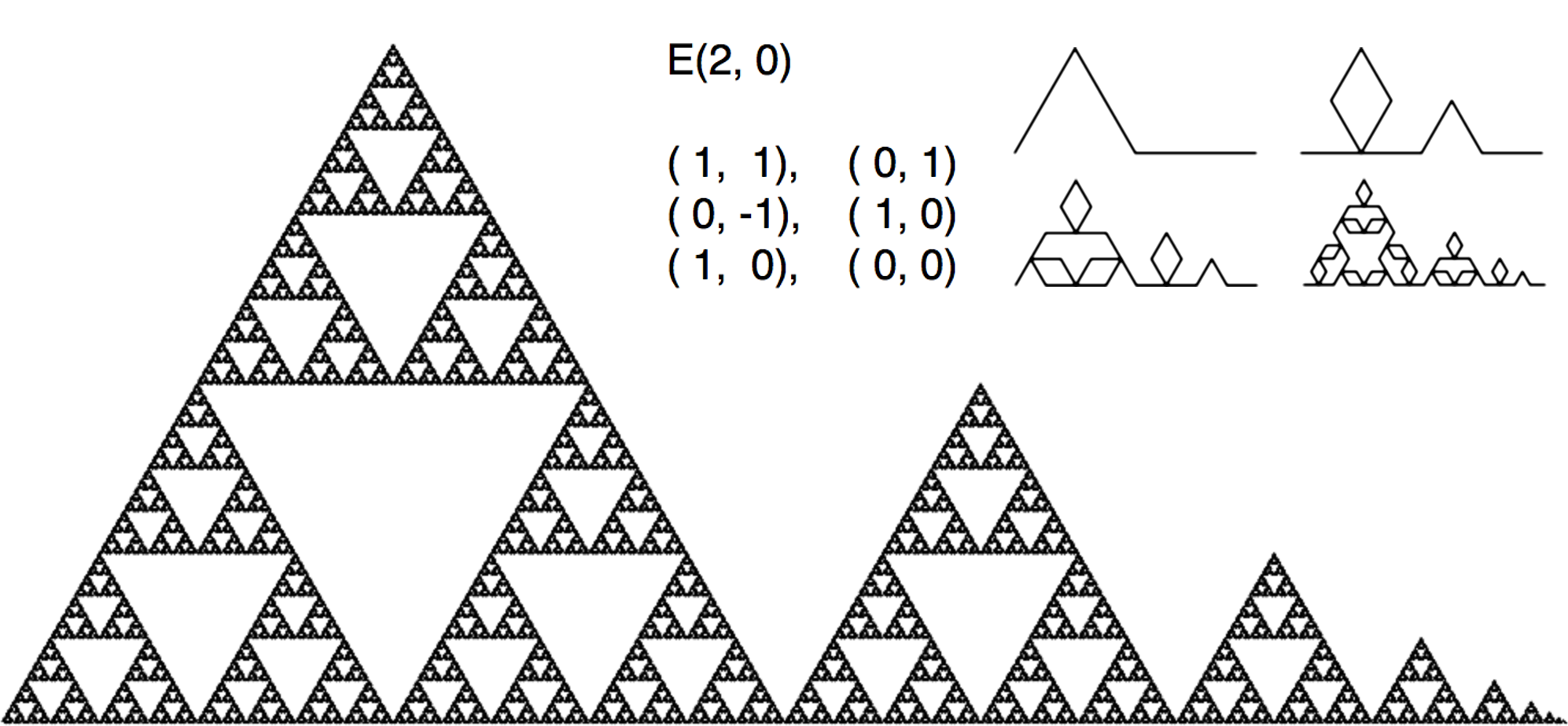Figure 5.11. The Sierpinski Family Dieter Steemann identified a variation of the Sierpinski Family that is self-avoiding , using an L-System (Figure 5.12). Might there be a way to describe his generator in the context of this taxonomy?Figure 5.12. Steemann’s variation of the Sierpinski Family Curves with straight-edge skins can also be found in families lying on the secondary axes (although regular polygon body shapes can only be found in families lying on the primary axis). Two such curves are shown in Figure 5.13.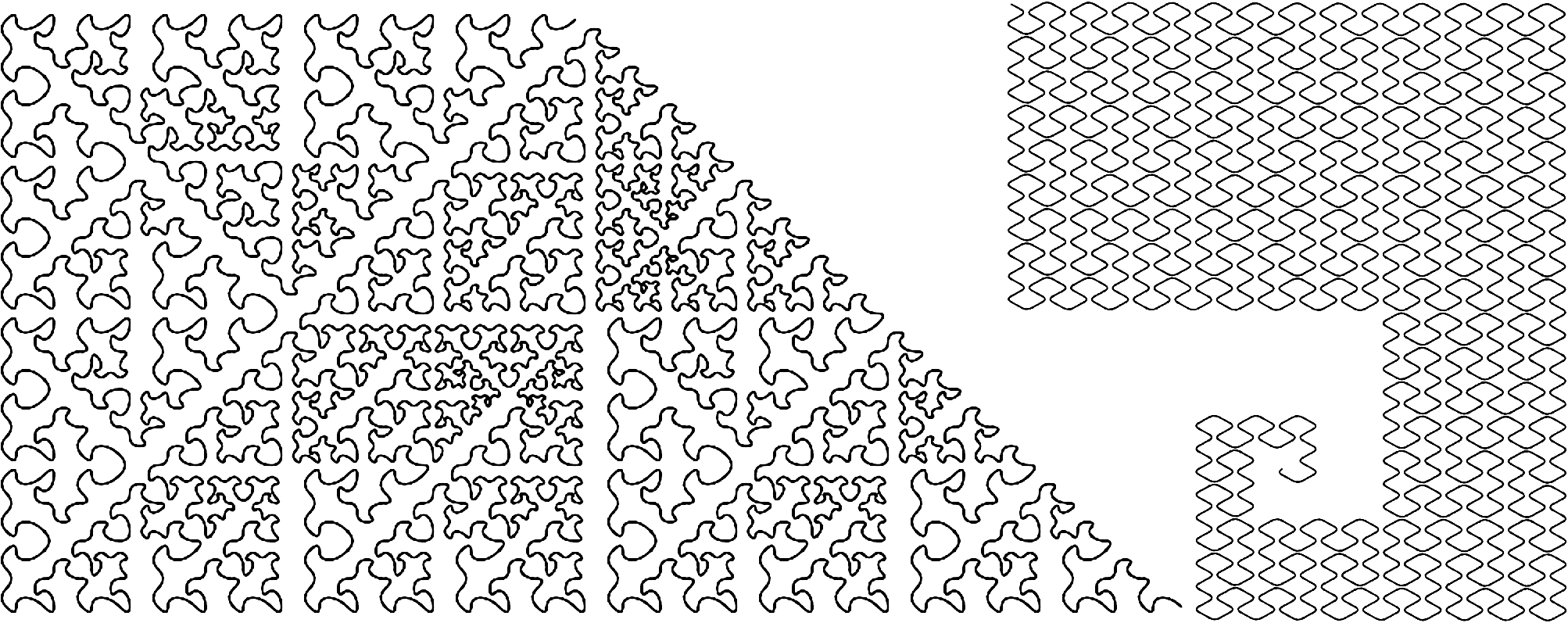Figure 5.13. Two curves from secondary axis families with straight skins (splined) Curves that lie on an axis can have transformations that include reflections, due to the bilateral symmetry about the axis. Families that do not lie on an axis have no straight-skinned curves (at least none have been found in the formulation of this taxonomy). The reason may have to do with the fact that there is no way to trace a straight path from the origin to the family integer that visits any lattice points along the way. Their skins require diversions from the axis of the spine. As an example, Figure 5.14 shows the six plane-filling curves of the G(2,1) family, with norm 5.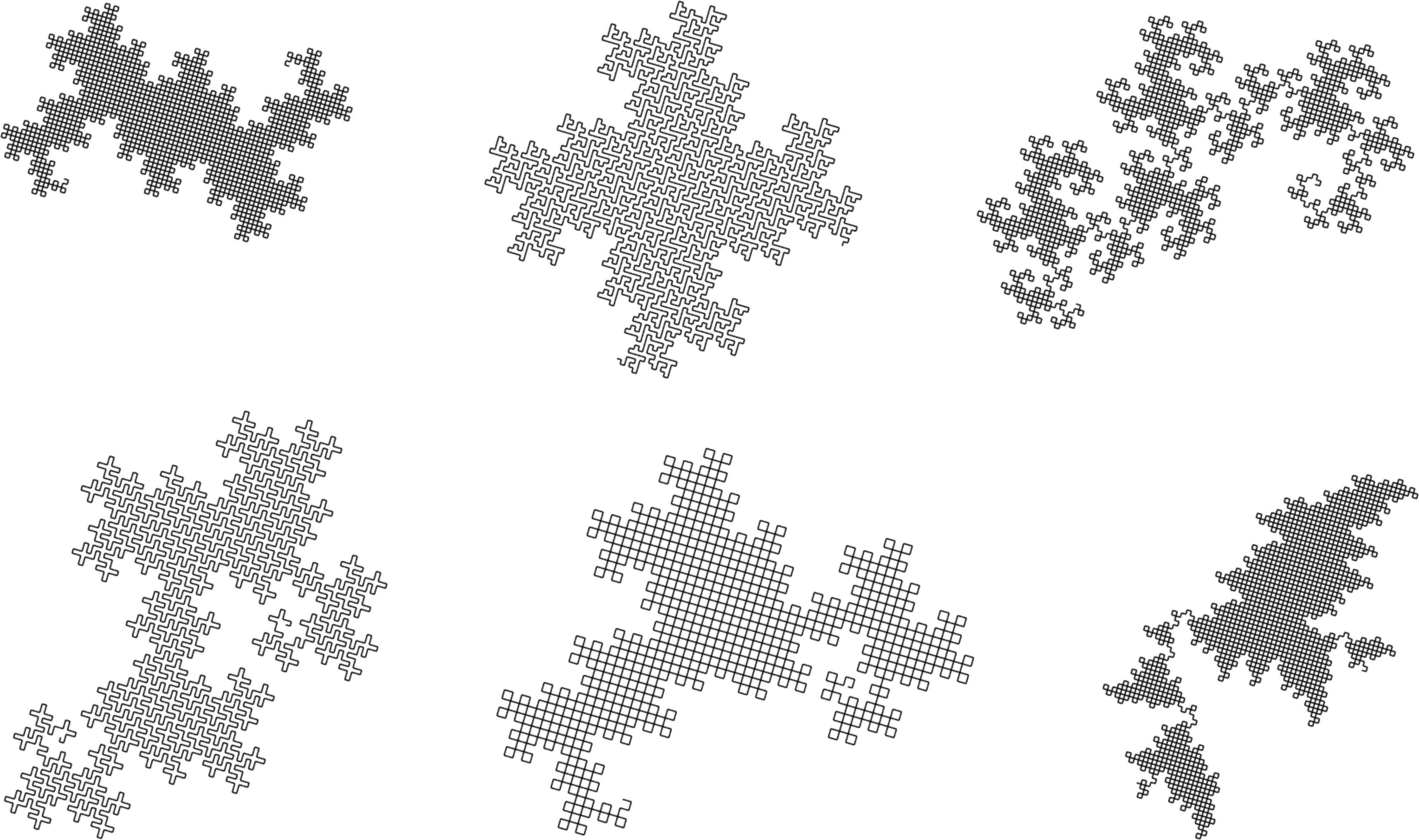Figure 5.14. Curves of the G(2,1) family This set includes the 5-Dragon and Mandelbrot’s Quartet. Similar skins can be detected in four of the curves. None of the skins are straight. Recall how the 5-Dragon generator cannot be reflected about its family integer because this would cause it to break out of the lattice. Now, let’s see what happens if we transform the 5-Dragon generator so that it corresponds to two families of norm 25. The generators shown at the left of Figure 5.15 are each made up of five integers of norm 5. This illustrates how two rotations of this generator can be associated with two different integers having norm 25.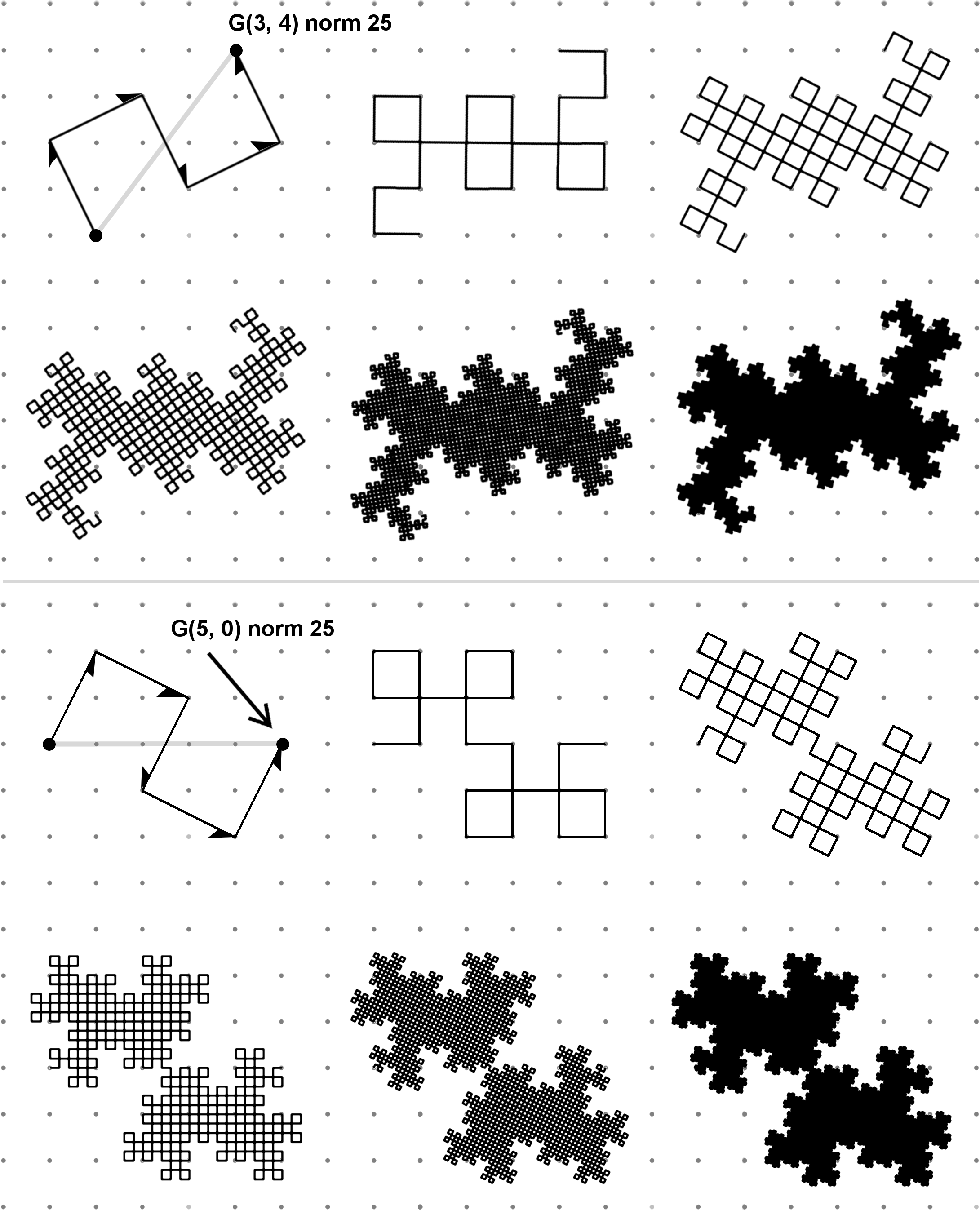Figure 5.15. 5-Dragon and inverted 5 Dragon shown in two families with norm 25 The integer G(3,4) shown at top is a perfect power whose root is G(2,1). The integer shown at bottom: G(5,0) is the product of G(2,1) and its conjugate G(2,-1). (The conjugate of a complex number is equal to the number with its imaginary component negated.) Multiplying a complex number by its conjugate always results in the product lying on the positive real axis. As a result, the segments in this generator can be reflected. Reflecting all of them results in a jagged butterfly shape, called “Inverted 5-Dragon”. Curiously, it appears that this generator allows only reflected segments. There are undoubtedly more such discoveries to be made, and considerations to be explored…perhaps relating to the fact that G(5,0) is not a perfect power, yet it is the product of conjugates (both having the same norm). With such discoveries beyond the current horizon, this taxonomy may need to be modified or enhanced. Twin Twins Not all curves that are members of axis-aligned families have straight skins. Let’s take a brief diversion and visit the Twindragon; a fractal shape with point-symmetry. It can be created by conjoining a HH dragon with a 180-rotated copy (Figure 5.16).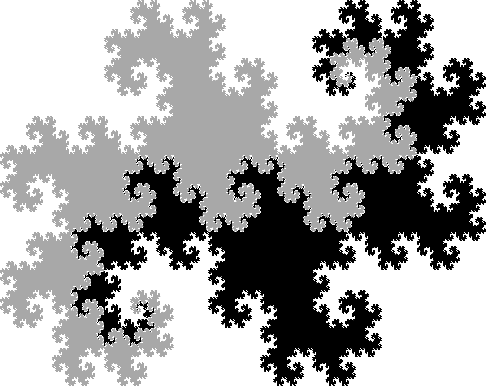Figure 5.16. Twindragon as a pertiling of two HH dragons Figure 5.17 shows three curves of the G(2,2) family with fractal skin. The first two are similar to the Twindragon. But unlike the Twindragon, the body shapes of these curves are comprised of different-sized “broken” Twindragons—kissing at fractally-distributed pinch-points. What accounts for these curious body shapes?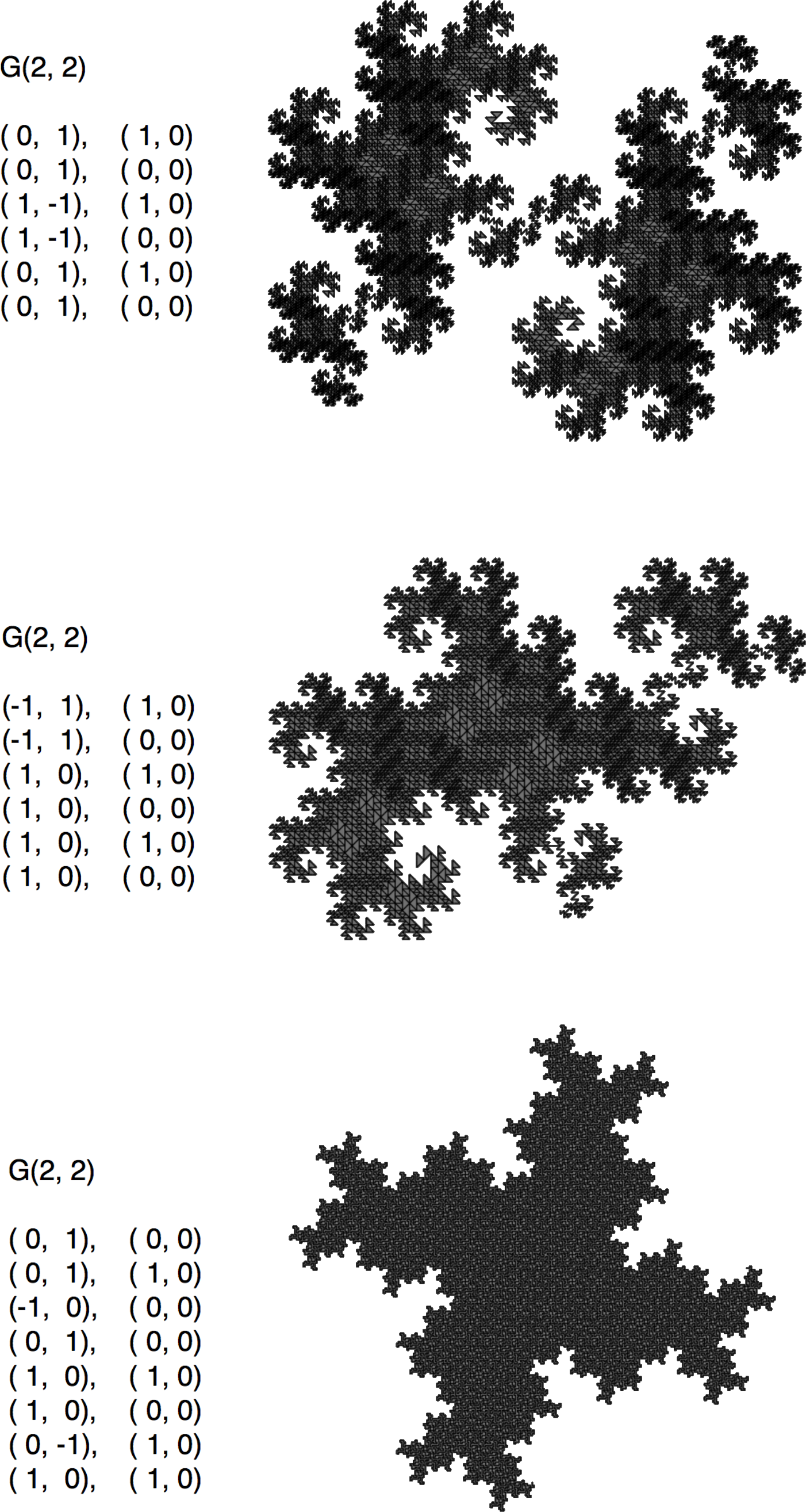Figure 5.17. Three curves of the G(2,2) family McKenna’s Curves Douglas McKenna developed categories for families of plane-filling curves based on square and triangular grids . Some of them are described here in the context of this taxonomy. Figure 5.18 shows the “E-curve” at top (generator and 2nd teragon). In this context, the E-curve can be described as a member of the G(5, 0) family, having norm 25. It is an axis-aligned, self-avoiding curve with a square body. The E-curve is the first in a series of self-avoiding curves that follow a certain logic for generator growth, shown at bottom. These are called “SquaRecurves”. The “order” of the generator is equal to the square root of the family norm (the Euclidean distance between generator’s endpoints, which lie on the real axis). Only odd-numbered orders admit curves in this series.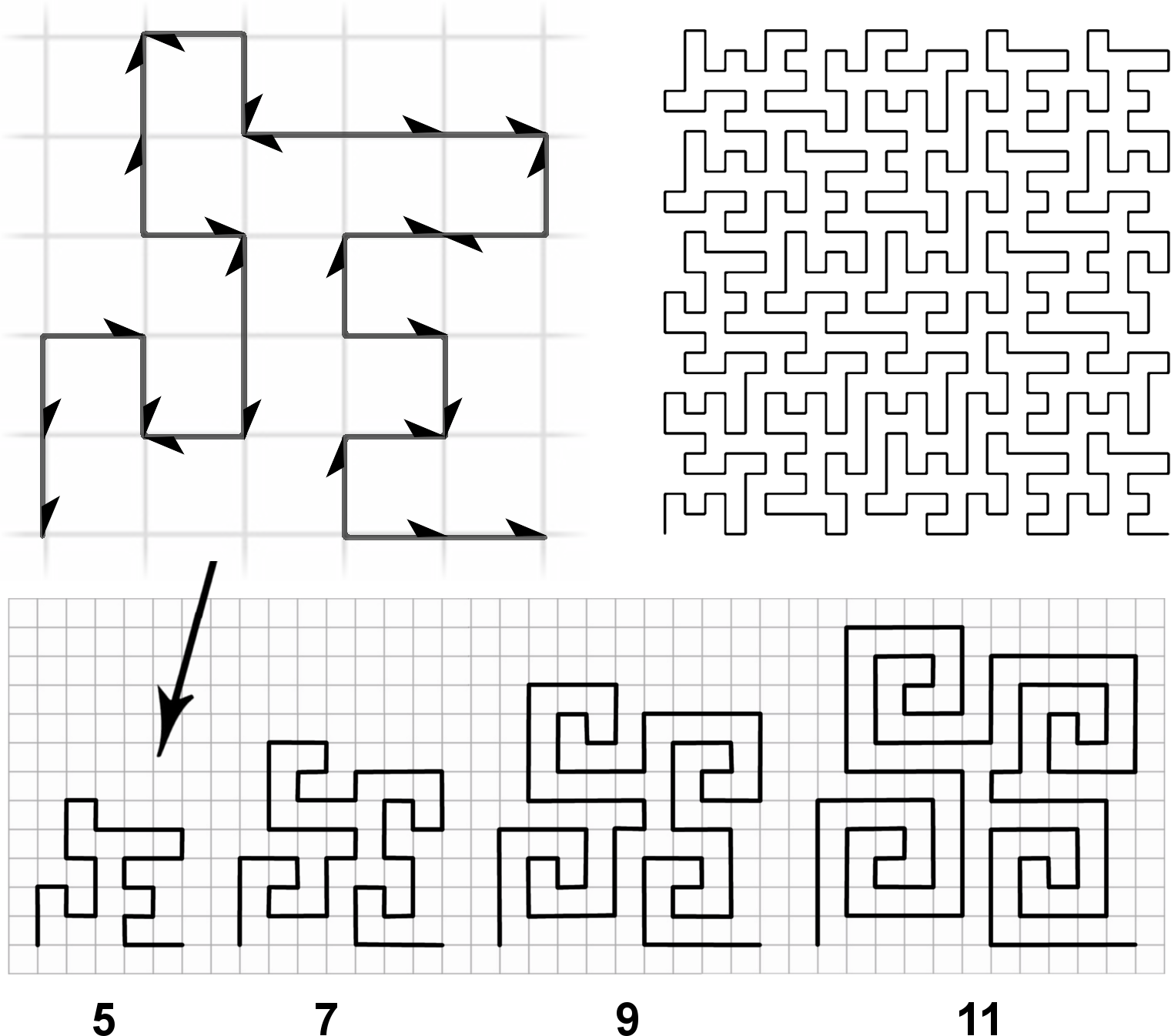Figure 5.18. The E curve (top) and the first few SquaRecurve generators Two other series of self-avoiding curves described by McKenna are shown in Figure 5.19. These include “Frenzies” (left) and “Eddies” (right). In this figure, the generators have been rotated and scaled to show how they fit within the family structure of this taxonomy. Notice that curve (a) is Mandelbrot’s Quartet, and that curve (b) is the G(3,2) self-avoider we saw earlier in Figure 2.18.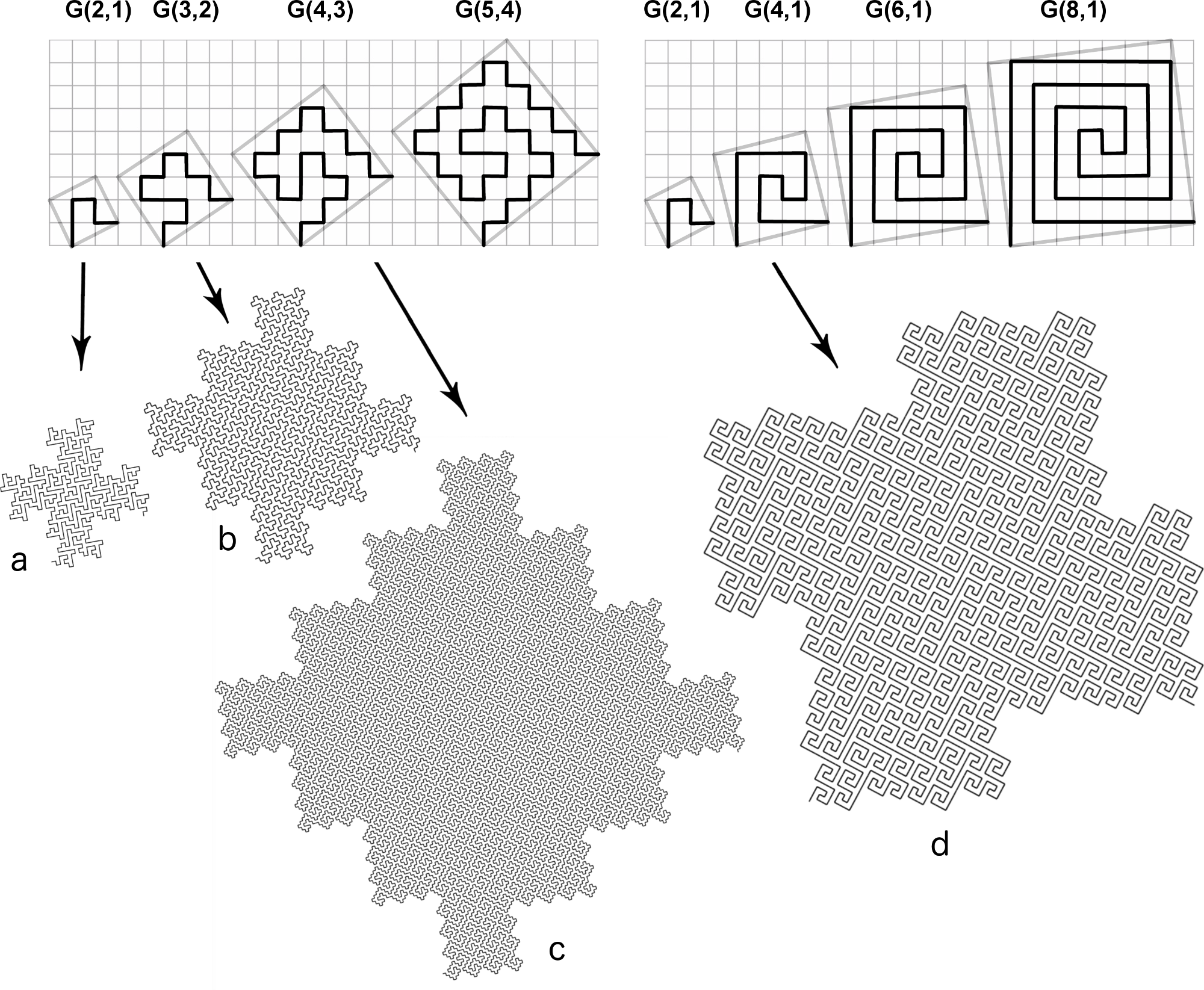Figure 5.19. Frenzies and Eddies Relation to Node-Replacement Curves Here’s a conjecture: every edge-replacement curve that permits monohedral tiling (i.e., all norms are identical) has an associated self-avoiding node-replacement curve, and the nodes correspond to the centers of the curve’s tiles. Below are some of these correlations. Peano/Hilbert The Hilbert curve was shown earlier as an example of a node-replacement curve. Figure 5.20 demonstrates how the Hilbert curve and the Peano sweep both undergo the same transform logic, as illustrated by the orientations of the tiles numbered 1 through 4.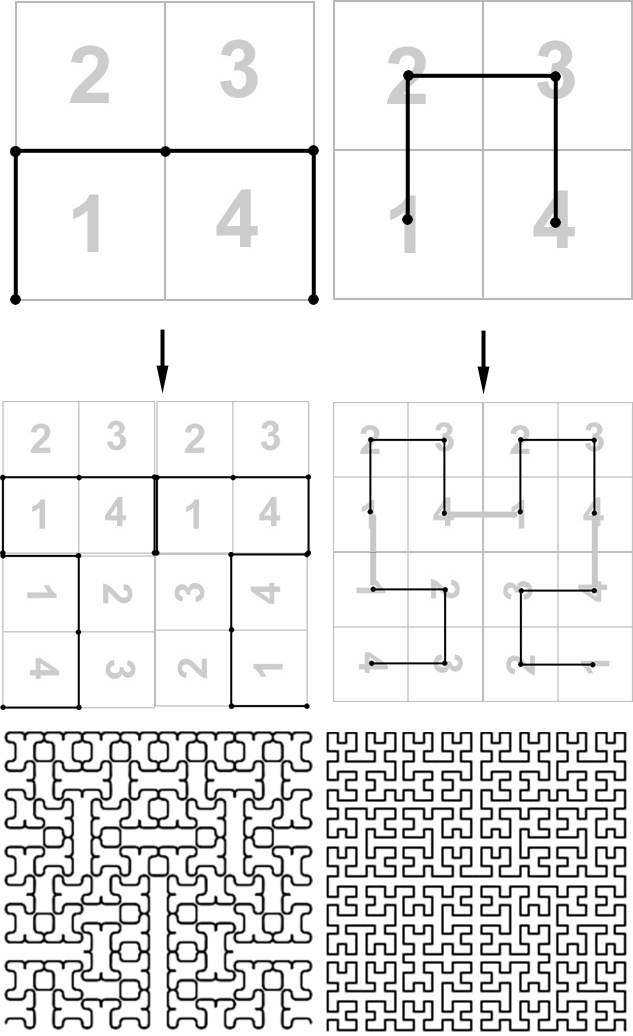Figure 5.20. Peano sweep (splined) compared to the Hilbert curve The tiling of the Peano sweep has the same scanning order as the nodes of the Hilbert curve. This comparison was also made by McKenna  and others. These transforms bring to mind the behaviors of turbulent fluids. Imagine an upward surge of fluid, as illustrated in Figure 5.21. As the dark fluid surges upward, the light-colored fluid around it gets pulled inward, creating a “neck”, as shown at top-right, and the dark fluid bifurcates at the top. A similar shape can be seen in the second teragons for the Peano sweep and the Hilbert curve.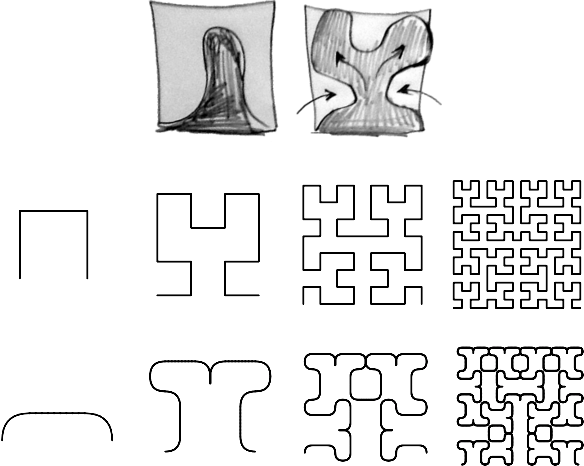Figure 5.21. fluid-like forms in the Hilbert curve and Peano sweep (splined) Eisenstein Variation of Peano/Hilbert The Peano sweep is a partially-self-avoiding curve, with an off-edge square tiling. There is an analogous curve in the Eisenstein domain: a triangular sweep with an off-edge triangle tiling. And, like the Peano sweep, this curve has a node-replacement counterpart, shown in Figure 5.22.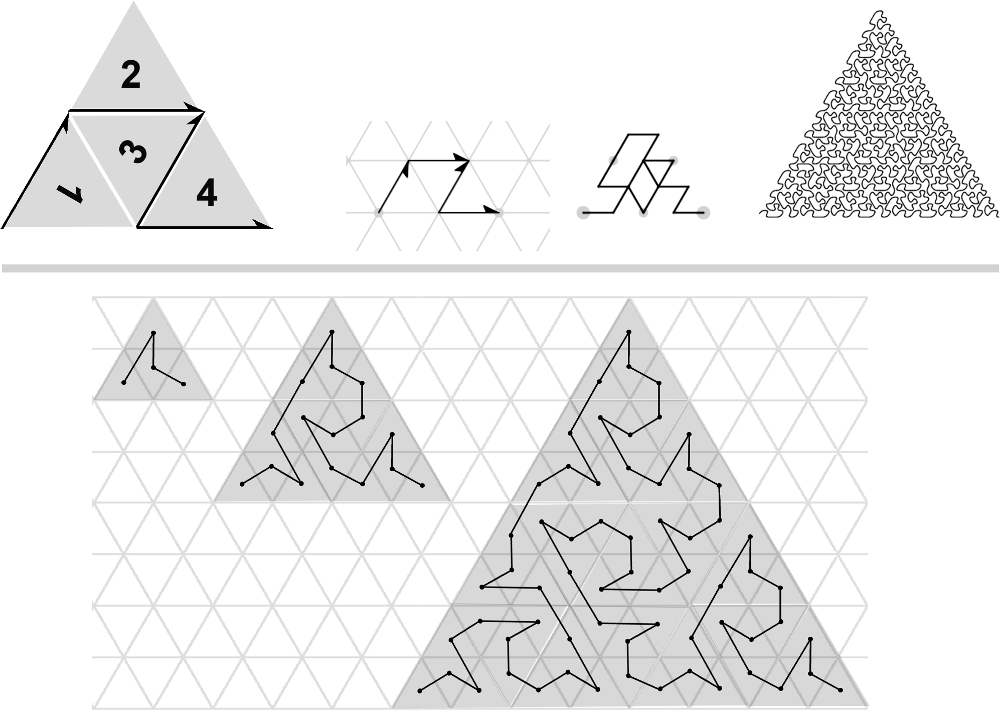Figure 5.22. A triangle sweep of the E(2,0) family, and a node-replacement counterpart Similar curves have been explored by Teachout , and Fiedorowicz . Node-Gosper Figure 5.23 shows a node-replacement version of the Gosper curve. The numerical order is plotted in the middle of each tile of the Gosper curve. These points are connected to form the node Gosper generator.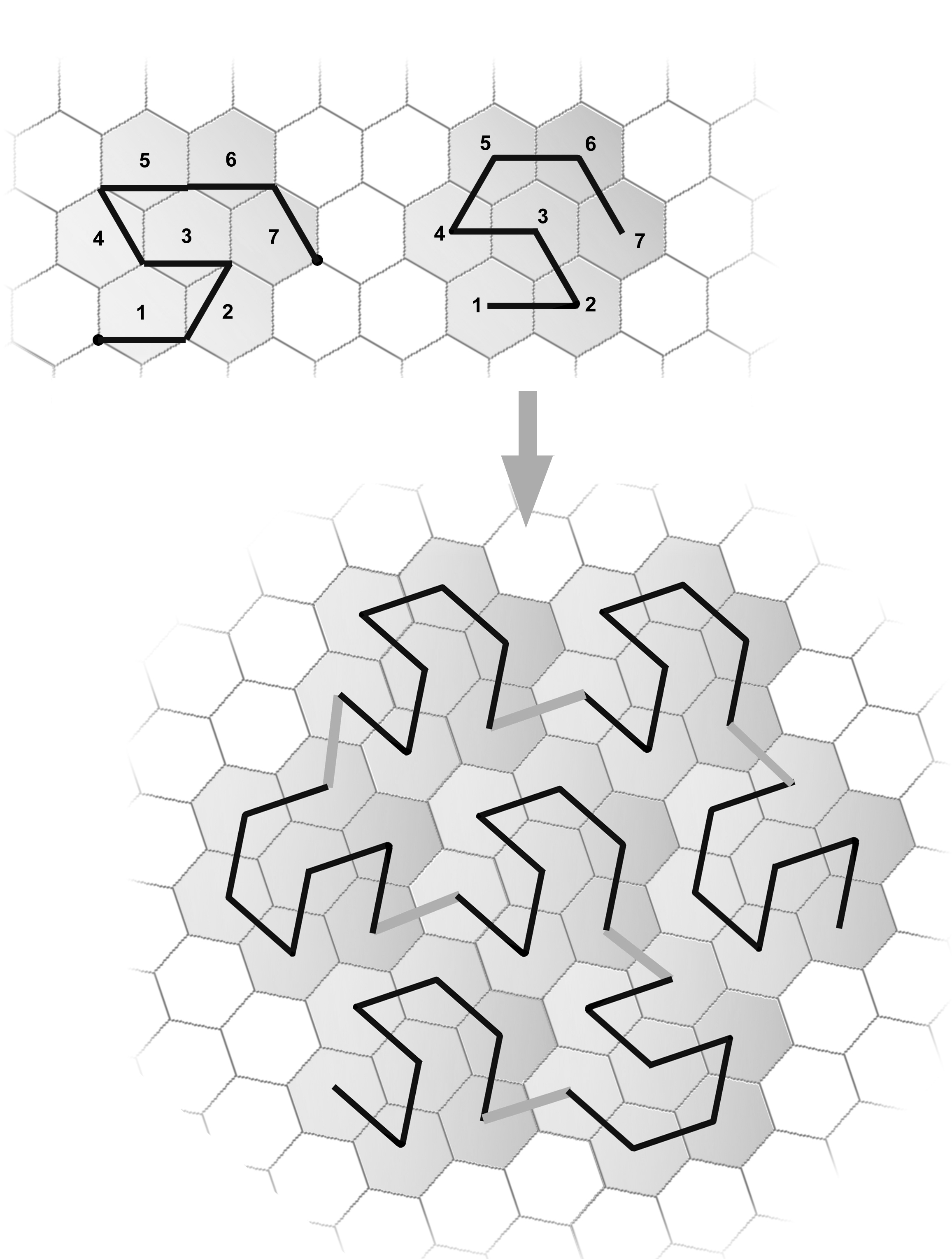Figure 5.23. Node Gosper Node-Quartet Figure 5.24 shows a node-replacement version of Mandelbrot’s Quartet.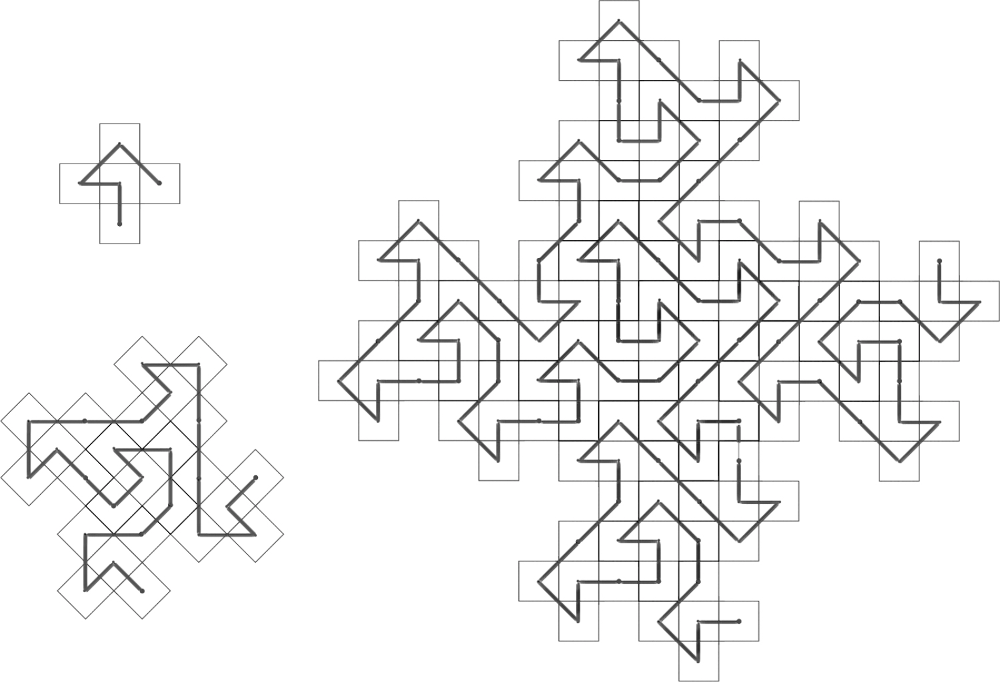Figure 5.24. The Node Quartet Node-Pinwheel The curve that corresponds to the pinwheel tiling described earlier has a node-replacement version, also suggested by Goucher .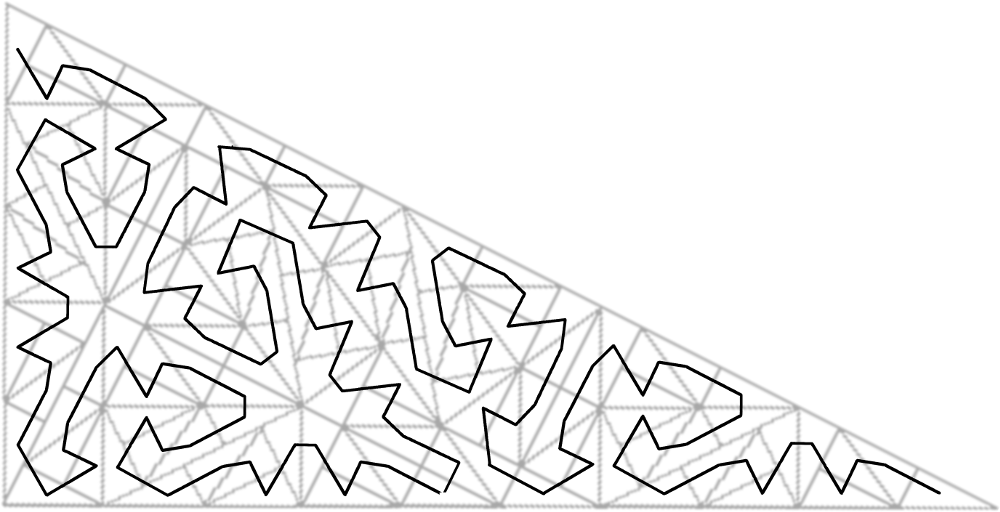Figure 5.25. Node-replacement curve on Pinwheel tiling Sierpinski/Cesàro The Sierpinski curve can be described as a node-replacement version of the Cesàro sweep. Figure 5.26 shows two variations of the Cesàro sweep. At the top of the figure is the version described by Mandelbrot, which is rotated here because it is treated as a member of the G(1,1) family. At the bottom is the G(1,1)2 version. In both versions, an off-edge right triangle is used to tile the area. The mid-points of the tiles are connected to create the Sierpinski curve. Notice how the 180-rotated segments in the G(1,1) version cause alternating teragon profiles, which do not appear in the G(1,1)2 version.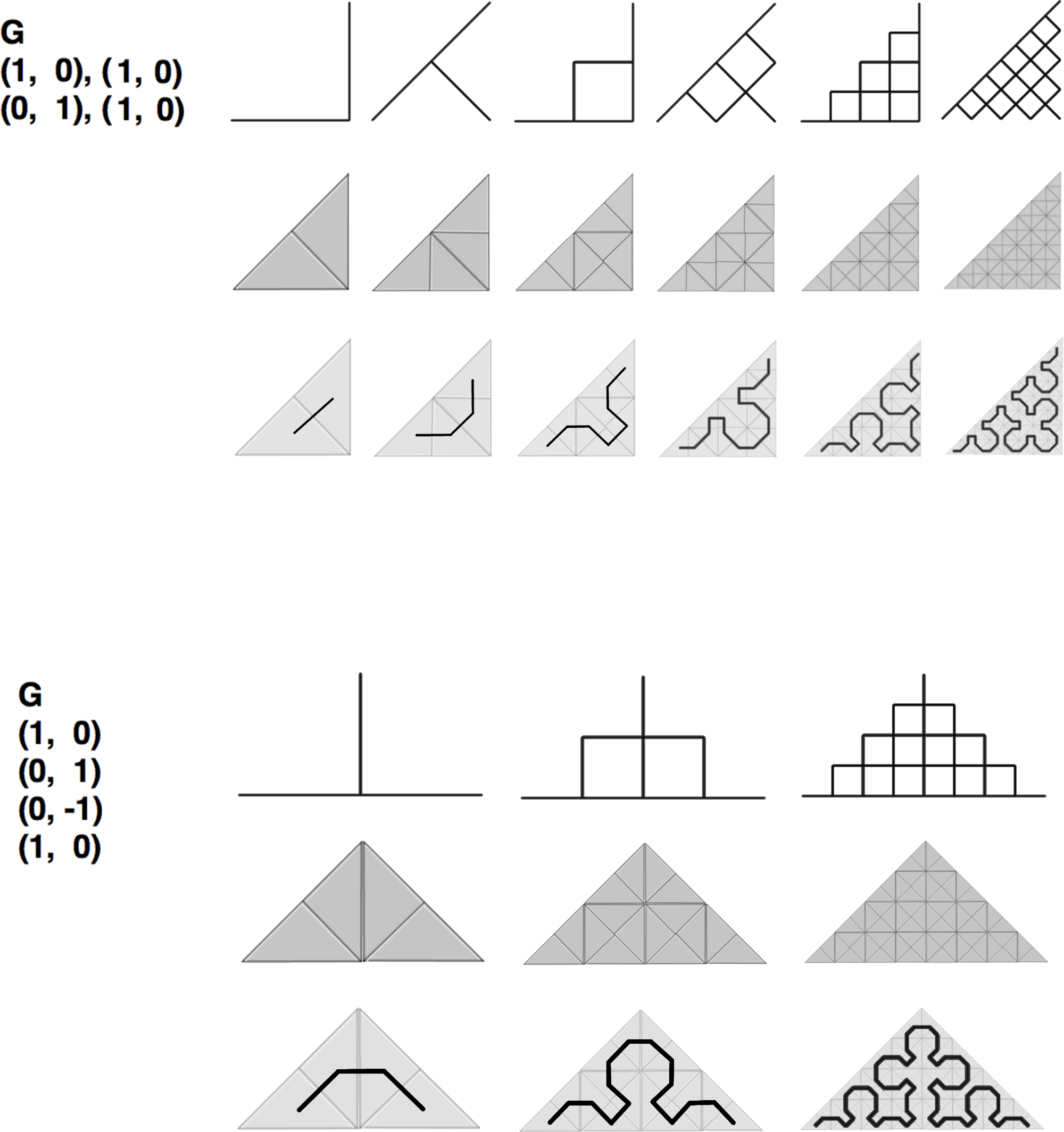Figure 5.26. Relationship between Cesàro sweep and Serpinski curve Node-Peano Two variations of the Peano curve provide another example of the relationship between node-replacement and edge-replacement (Figure 5.27). Both of their generators occupy a 3x3 grid, and they map to each other by way of a 45-degree rotation (bottom row).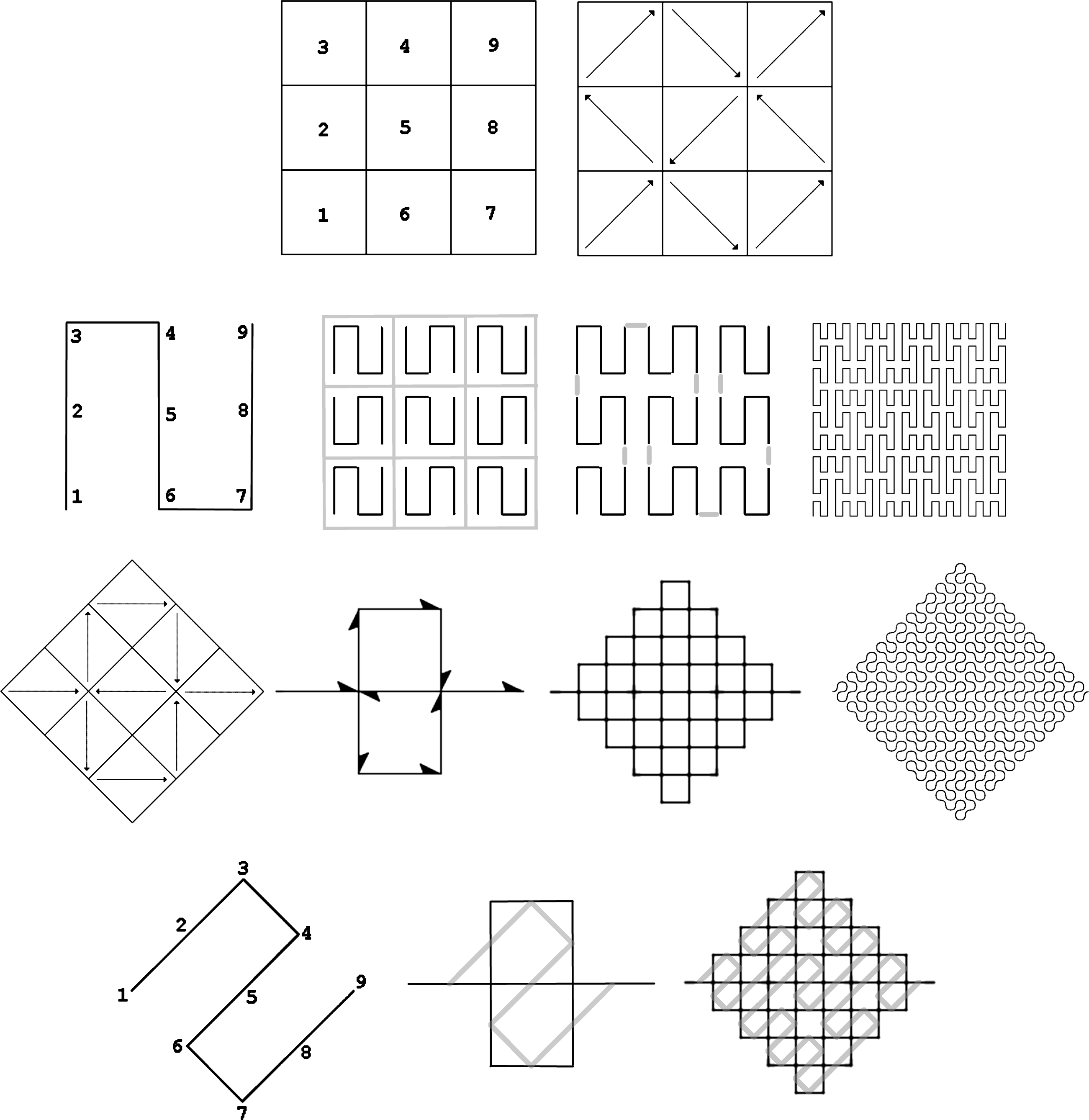Figure 5.27. Peano curve: tiling, node-replacement, edge-replacement, and overlap Alexander Bogomolny identified a set of variations of the Peano curve by applying reflections to the tiles of the generator . These reflections would have no visual effect in the edge-replacement version, since the generator is symmetrical about the segment. But they are easily seen in the node-replacement version. Bogomolny identified a total of 272 variations, given all combinations of reflections. A teragon of one such variation is shown in Figure 5.28.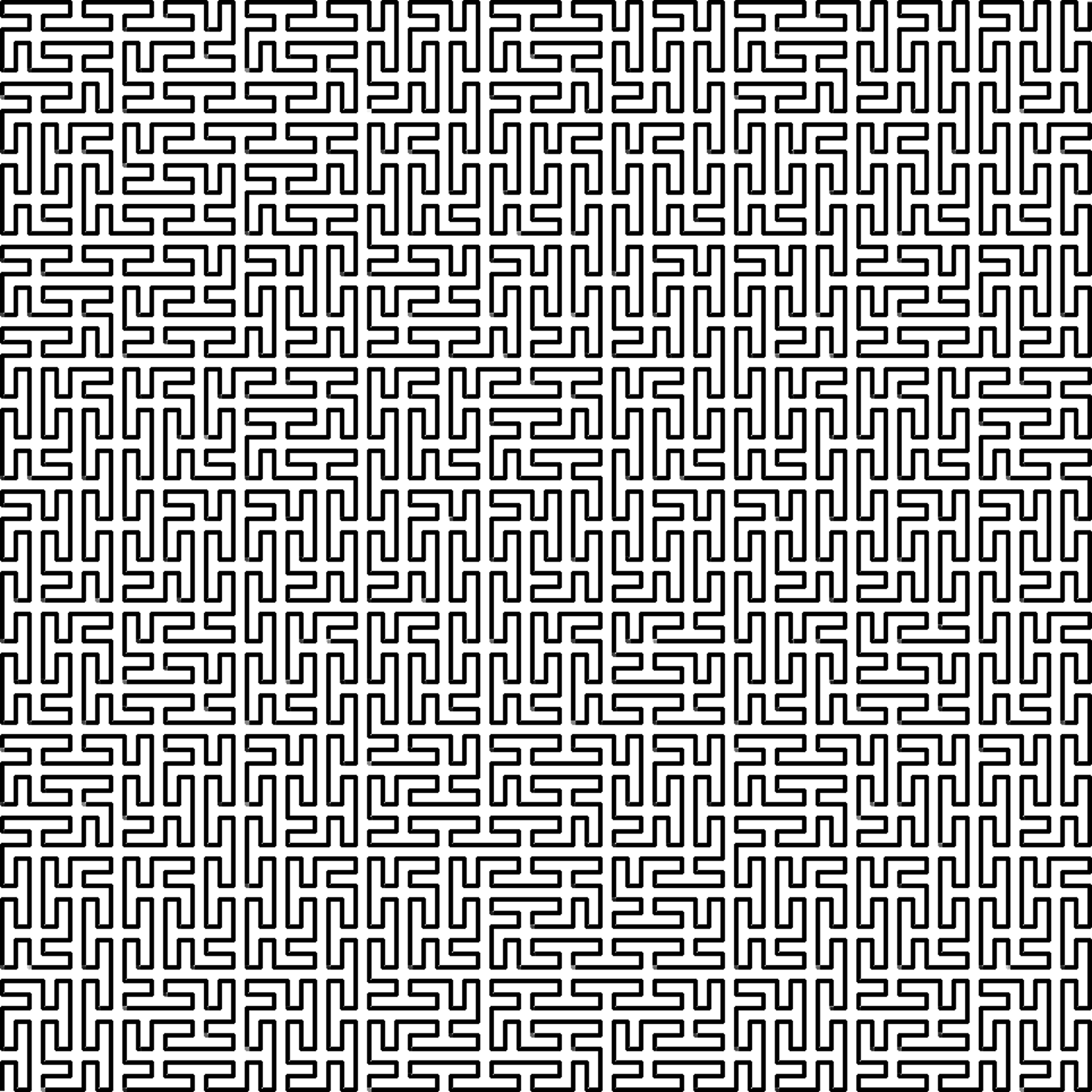Figure 5.28. One of 272 variations of the node-replacement Peano curve, using reflections Within the same family (G(3,0)), a variation of the Wunderlich curve called the “Peano-Meander curve” (a node-replacement curve) has a complementary edge-replacement curve of the G(3,0) family (bottom of Figure 5.29). The ordering of its nine tiles allows nine continuous segments to be drawn, starting at G(0, 0,) and ending at G(3, 0), such that each segment coincides with a unique tile edge. Similar to the Peano sweep, this curve’s generator has overlapping segments (5th and 6th).Figure 5.29. A Wunderlich curve and its associated edge-replacement curve As proposed before, if a monohedral tiling can be associated with an edge-replacement curve, then it is possible to create a complimentary node-replacement curve by connecting the midpoints of the tiles. But it seems the reverse is not always true. Some node-replacement curves (e.g., the Moore curve  and the Z-order (Lebesgue) curve—Figure 5.30) do not have associated edge-replacement curves, because of the spatial ordering of their tiles. In the Moore curve (top), which consists of 4 scaled-down, rotated versions of the Hilbert curve, the first and last tiles are adjacent. In the Z-order curve (bottom), there are consecutive nodes that jump between non-adjacent tiles.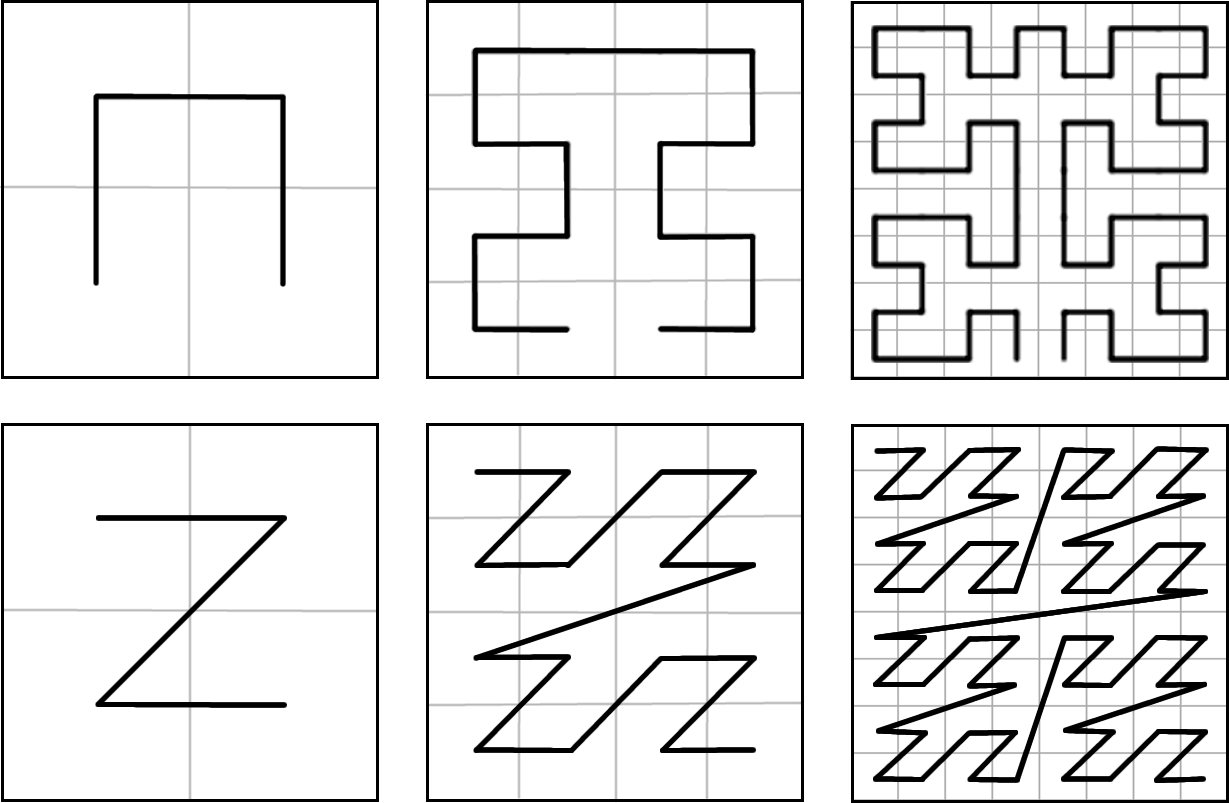Figure 5.30. The Moore and Z-order curves have no associated edge-replacement curvesFigure 5.31. Stylized curve with dimension < 2 that partially fills the Koch snowflakeThe Family Tree of Fractal Curves A taxonomy of plane-filling curves using complex integer lattices by Jeffrey Ventrella 1 Introduction 2 Taxonomy 3 Iteration 4 Morphology 5 Family Resemblances 6 Undiscovered Curiosities 7 References